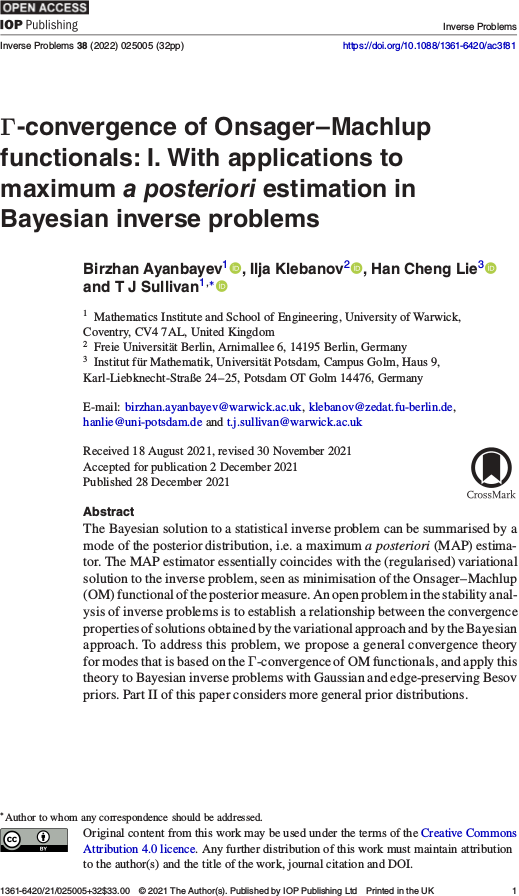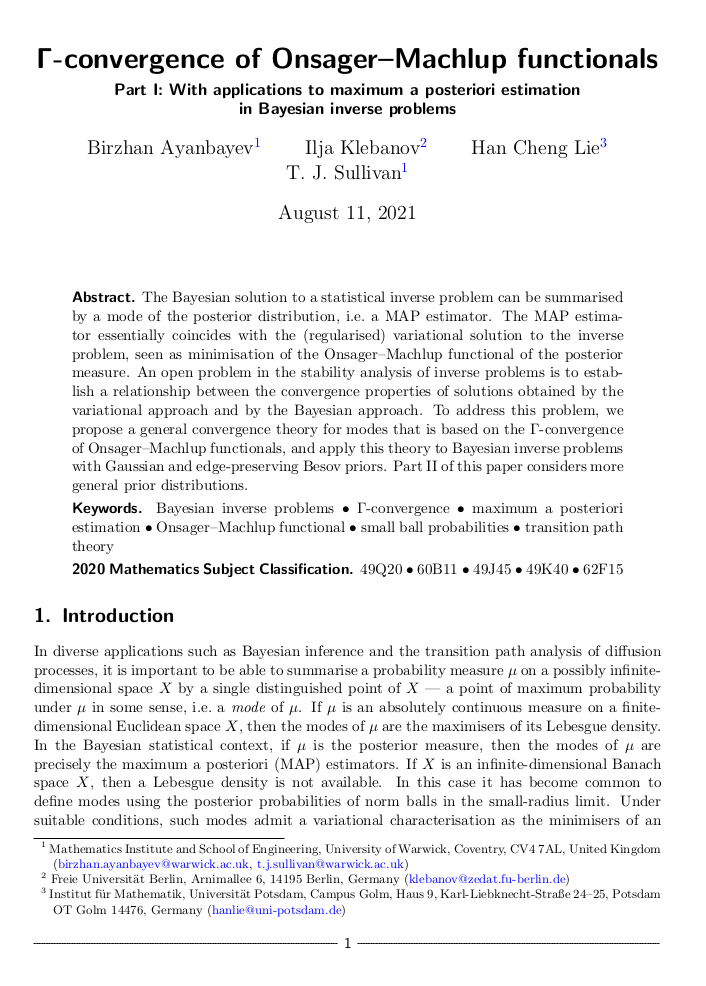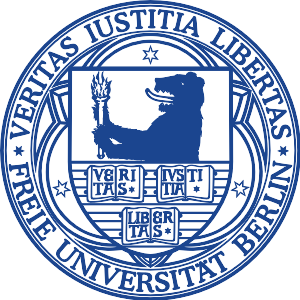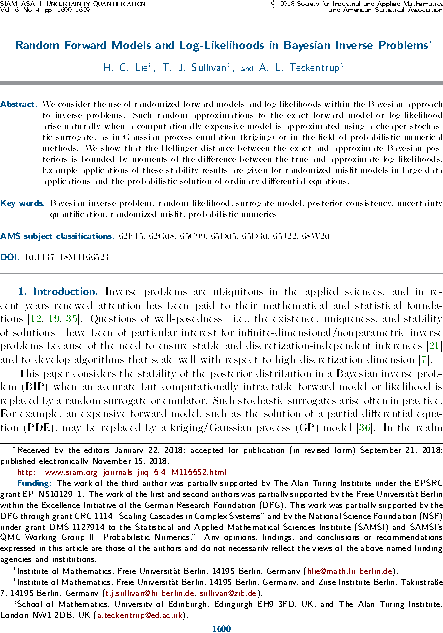# Tim Sullivan

### #inverse-problems

Clear Search### Γ-convergence of Onsager-Machlup functionals in Inverse Problems

The articles “Γ-convergence of Onsager–Machlup functionals” (“I. With applications to maximum a posteriori estimation in Bayesian inverse problems” and “II. Infinite product measures on Banach spaces”) by Birzhan Ayanbayev, Ilja Klebanov, Han Cheng Lie, and myself have just appeared in their final form in the journal Inverse Problems.

The purpose of this work is to address a long-standing issue in the Bayesian approach to inverse problems, namely the joint stability of a Bayesian posterior and its modes (MAP estimators) when the prior, likelihood, and data are perturbed or approximated. We show that the correct way to approach this problem is to interpret MAP estimators as global weak modes in the sense of Helin and Burger (2015), which can be identified as the global minimisers of the Onsager–Machlup functional of the posterior distribution, and hence to provide a convergence theory for MAP estimators in terms of Γ-convergence of these Onsager–Machlup functionals. It turns out that posterior Γ-convergence can be assessed in a relatively straightforward manner in terms of prior Γ-convergence and continuous convergence of potentials (negative log-likelihoods). Over the two parts of the paper, we carry out this programme both in generality and for specific priors that are commonly used in Bayesian inverse problems, namely Gaussian and Besov priors (Lassas et al., 2009; Dashti et al., 2012).

B. Ayanbayev, I. Klebanov, H. C. Lie, and T. J. Sullivan. “Γ-convergence of Onsager–Machlup functionals: I. With applications to maximum a posteriori estimation in Bayesian inverse problems.” Inverse Problems 38(2):025005, 32pp., 2022. doi:10.1088/1361-6420/ac3f81

Abstract (Part I). The Bayesian solution to a statistical inverse problem can be summarised by a mode of the posterior distribution, i.e. a MAP estimator. The MAP estimator essentially coincides with the (regularised) variational solution to the inverse problem, seen as minimisation of the Onsager–Machlup functional of the posterior measure. An open problem in the stability analysis of inverse problems is to establish a relationship between the convergence properties of solutions obtained by the variational approach and by the Bayesian approach. To address this problem, we propose a general convergence theory for modes that is based on the Γ-convergence of Onsager–Machlup functionals, and apply this theory to Bayesian inverse problems with Gaussian and edge-preserving Besov priors. Part II of this paper considers more general prior distributions.

B. Ayanbayev, I. Klebanov, H. C. Lie, and T. J. Sullivan. “Γ-convergence of Onsager–Machlup functionals: II. Infinite product measures on Banach spaces.” Inverse Problems 38(2):025006, 35pp., 2022. doi:10.1088/1361-6420/ac3f82

Abstract (Part II). We derive Onsager–Machlup functionals for countable product measures on weighted $$\ell^{p}$$ subspaces of the sequence space $$\mathbb{R}^\mathbb{N}$$. Each measure in the product is a shifted and scaled copy of a reference probability measure on $$\mathbb{R}$$ that admits a sufficiently regular Lebesgue density. We study the equicoercivity and Γ-convergence of sequences of Onsager–Machlup functionals associated to convergent sequences of measures within this class. We use these results to establish analogous results for probability measures on separable Banach or Hilbert spaces, including Gaussian, Cauchy, and Besov measures with summability parameter $$1 \leq p \leq 2$$. Together with Part I of this paper, this provides a basis for analysis of the convergence of maximum a posteriori estimators in Bayesian inverse problems and most likely paths in transition path theory.

Published on Wednesday 5 January 2022 at 12:00 UTC #publication #inverse-problems #modes #map-estimators #ayanbayev #klebanov #lie### Γ-convergence of Onsager-Machlup functionals

Birzhan Ayanbayev, Ilja Klebanov, Han Cheng Lie, and I have just uploaded preprints of our work “Γ-convergence of Onsager–Machlup functionals” to the arXiv; this work consists of two parts, “Part I: With applications to maximum a posteriori estimation in Bayesian inverse problems” and “Part II: Infinite product measures on Banach spaces”.

The purpose of this work is to address a long-standing issue in the Bayesian approach to inverse problems, namely the joint stability of a Bayesian posterior and its modes (MAP estimators) when the prior, likelihood, and data are perturbed or approximated. We show that the correct way to approach this problem is to interpret MAP estimators as global weak modes in the sense of Helin and Burger (2015), which can be identified as the global minimisers of the Onsager–Machlup functional of the posterior distribution, and hence to provide a convergence theory for MAP estimators in terms of Γ-convergence of these Onsager–Machlup functionals. It turns out that posterior Γ-convergence can be assessed in a relatively straightforward manner in terms of prior Γ-convergence and continuous convergence of potentials (negative log-likelihoods). Over the two parts of the paper, we carry out this programme both in generality and for specific priors that are commonly used in Bayesian inverse problems, namely Gaussian and Besov priors (Lassas et al., 2009; Dashti et al., 2012).

Abstract (Part I). The Bayesian solution to a statistical inverse problem can be summarised by a mode of the posterior distribution, i.e. a MAP estimator. The MAP estimator essentially coincides with the (regularised) variational solution to the inverse problem, seen as minimisation of the Onsager–Machlup functional of the posterior measure. An open problem in the stability analysis of inverse problems is to establish a relationship between the convergence properties of solutions obtained by the variational approach and by the Bayesian approach. To address this problem, we propose a general convergence theory for modes that is based on the Γ-convergence of Onsager–Machlup functionals, and apply this theory to Bayesian inverse problems with Gaussian and edge-preserving Besov priors. Part II of this paper considers more general prior distributions.

Abstract (Part II). We derive Onsager–Machlup functionals for countable product measures on weighted $$\ell^{p}$$ subspaces of the sequence space $$\mathbb{R}^\mathbb{N}$$. Each measure in the product is a shifted and scaled copy of a reference probability measure on $$\mathbb{R}$$ that admits a sufficiently regular Lebesgue density. We study the equicoercivity and Γ-convergence of sequences of Onsager–Machlup functionals associated to convergent sequences of measures within this class. We use these results to establish analogous results for probability measures on separable Banach or Hilbert spaces, including Gaussian, Cauchy, and Besov measures with summability parameter $$1 \leq p \leq 2$$. Together with Part I of this paper, this provides a basis for analysis of the convergence of maximum a posteriori estimators in Bayesian inverse problems and most likely paths in transition path theory.

Published on Wednesday 11 August 2021 at 12:00 UTC #preprint #inverse-problems #modes #map-estimators #ayanbayev #klebanov #lie### Postdoc position: Analysis of MAP estimators

There is still an opening for a full-time two-year postdoctoral research position in the UQ group at the Freie Universität Berlin. This position will be associated to the project “Analysis of maximum a posteriori estimators: Common convergence theories for Bayesian and variational inverse problems” funded by the DFG.

This project aims to advance the state of the art in rigorous mathematical understanding of MAP estimators in infinite-dimensional statistical inverse problems. In particular, the research in this project will connect the “small balls” approach of Dashti et al. (2013) to the calculus of variations and hence properly link the variational and fully Bayesian points of view on inverse problems. This is an exciting opportunity for someone well-versed in the calculus of variations and tools such as Γ-convergence to make an impact on fundamental questions of non-parametric statistics and inverse problems or, vice versa, for someone with a statistical inverse problems background to advance the rigorous state of the art for such methods.

Prospective candidates are encouraged to contact me with informal enquiries. Formal applications are to be sent by post or email, by 23 December 2019, under the heading MAP-Analysis, and should include a cover letter, a scientific CV including list of publications and research statement, and the contact details of two professional references.

Published on Monday 25 November 2019 at 08:00 UTC #group #job #fu-berlin #inverse-problems #dfg #map-estimators### Course in Inverse Problems at FU Berlin

This Summer Semester 2019, I will offer a course in the mathematics of Inverse Problems at the Freie Universität Berlin. The course load will be 2+2 SWS and the course will be a valid selection for the Numerik IV and Stochastic IV modules.

Inverse problems, meaning the recovery of parameters or states in a mathematcial model that best match some observed data, are ubiquitous in applied sciences. This course will provide an introduction to the deterministic (variational) and stochastic (Bayesian) theories of inverse problems in function spaces.

#### Course Contents

1. Examples of inverse problems in mathematics and physical sciences
2. Preliminaries from functional analysis
3. Preliminaries from probability theory
4. Linear inverse problems and variational regularisation
5. Bayesian regularisation of inverse problems
6. Monte Carlo methods for Bayesian problems

For further details see the KVV page.

Published on Monday 8 April 2019 at 09:00 UTC #fub #inverse-problems### Random Bayesian inverse problems in JUQ

The article “Random forward models and log-likelihoods in Bayesian inverse problems” by Han Cheng Lie, Aretha Teckentrup, and myself has now appeared in its final form in the SIAM/ASA Journal on Uncertainty Quantification, volume 6, issue 4. This paper considers the effect of approximating the likelihood in a Bayesian inverse problem by a random surrogate, as frequently happens in applications, with the aim of showing that the perturbed posterior distribution is close to the exact one in a suitable sense. This article considers general randomisation models, and thereby expands upon the previous investigations of Stuart and Teckentrup (2018) in the Gaussian setting.

H. C. Lie, T. J. Sullivan, and A. L. Teckentrup. “Random forward models and log-likelihoods in Bayesian inverse problems.” SIAM/ASA Journal on Uncertainty Quantification 6(4):1600–1629, 2018. doi:10.1137/18M1166523

Abstract. We consider the use of randomised forward models and log-likelihoods within the Bayesian approach to inverse problems. Such random approximations to the exact forward model or log-likelihood arise naturally when a computationally expensive model is approximated using a cheaper stochastic surrogate, as in Gaussian process emulation (kriging), or in the field of probabilistic numerical methods. We show that the Hellinger distance between the exact and approximate Bayesian posteriors is bounded by moments of the difference between the true and approximate log-likelihoods. Example applications of these stability results are given for randomised misfit models in large data applications and the probabilistic solution of ordinary differential equations.

Published on Monday 10 December 2018 at 12:00 UTC #publication #bayesian #inverse-problems #juq #prob-num #lie #teckentrup